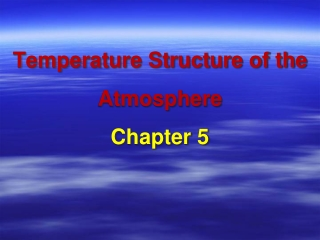DownloadDownload PresentationTemperature Structure of the Atmosphere Chapter 5

# Temperature Structure of the Atmosphere Chapter 5

Télécharger la présentation## Temperature Structure of the Atmosphere Chapter 5

- - - - - - - - - - - - - - - - - - - - - - - - - - - E N D - - - - - - - - - - - - - - - - - - - - - - - - - - -
##### Presentation Transcript

1. Temperature Structure of the AtmosphereChapter 5

2. Greenhouse effect is powered by lapse rate. • Has to be cold higher in atm. • Have to add in processes not found in layer model • Need moist convection

3. T structure of atm is coupled to T of ground by convection. • Warm air rises and carries heat • As it expands, it cools. • Leads to dec. in T with inc. altitude. • If atm were incompressible, like water, there would be little change in T with altitude. • no gh effect because amount of outgoing IR would be the same whether it came from the ground or high in the atm.

4. Typical T and P of atm as a function of altitude • Troposhpere - 90% of gas molecules, weather, climate models • Stratosphere • More layers above…. • P nonlinear with altitude (exponential)

5. Fig. 5.2 - P and depth are linear • Water is not compressible • So it increases linearly with depth

6. Adiabatic expansion • Lapse rate - T dec. w/altitude • Adiabatic - air heats/cools by expansion/compression, not due to T of air around it. • Dry vs. moist

7. Sinking = compressing = heating

8. Effect of water on the T profile of atm. • Air cools as it rises, water vapor condenses to droplets, releases LH. • So, moist adiabatis less than dry.

9. Water vapor & latent heat • 3 phases of water • Vapor = liquid + heat • Latent heat = energy tied up in water vapor • Sensible heat – what we measure with a thermometer • Condensation - LH SH

10. Convection • Carries heat (along with radiation and conduction) • Transfers heat from equator to poles • Occurs in fluids (liquid & gas) • Driven by heating from below • Molecules move energetically, fluid expands, density decreases. Heating from below Stable

11. Convection in the layer model • Layer model from chapter 3 didn’t have convection. • T of atm. layer decreases with altitude • But, in earlier model, heat is only carried upward by radiation. • Need to add convection to the model.

12. C 3000 m B 0 m A D 0 m DALR = 10 deg C/Km Point A: T = 30 deg C Point B: T = 20 deg C SALR = 6 deg C/Km Point C: T = 8 deg C Point D: T = 38 deg C 1000 m

13. Convection in the layer model If we add convection to the layer model then we have to add another set of heat arrows.

14. Lapse rate & gh effect • The steeper the lapse rate, the stronger the gh effect. • If the atm were incompressible , like water, and convection maintained a uniform T w/altitude then there would be no gh effect. • Raise CO2 in atm raises the altitude in the atm where IR escapes to space. A to B - Inc. amount of gh gas. IR to space at a higher altitude (new skin altitude). But, the skin T stays the Same (red line). Inc. in skin T altitude -- inc. ground T A B A

15. Take Home Points • Air in the upper troposphere is colder due to moist convection. • Sunlight heats surface, air rises and cools • P decreases with altitude • T decreases as a gas expands • LH is released as SH as water vapor condenses. • Lapse rate is controlled by the moist adiabat. • Strength of gh effect depends on the lapse rate. • Need another set of arrows in our model!!